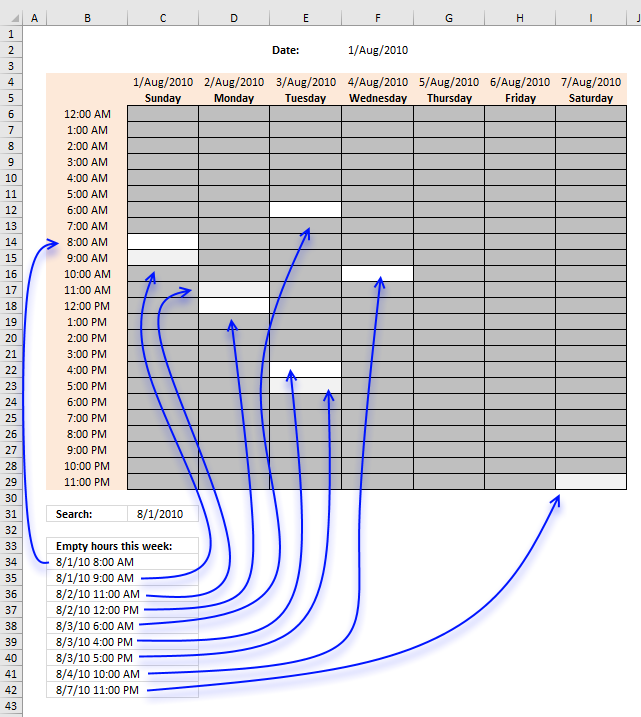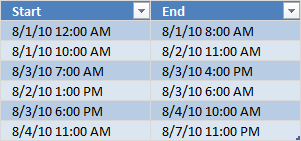Author: Oscar Cronquist Article last updated on December 28, 2018The image above demonstartes an array formula in cell B34 that extracts empty hours in a weekly calendar. I have created some random time ranges located in an Excel defined table. We are going to use these time ranges to extract empty hours between ranges.I have reused the same weekly schedule template as in this post: Highlight specific time ranges in a weekly schedule As you can see in the top picture, I have applied conditional formatting to "highlight" the random date/time ranges I created earlier.

You can easily spot empty hours but how do you filter all the empty hours for this week? The following array formula demonstrates how:

Array formula in B34:

=SMALL(IF(FREQUENCY(IF((\$C\$31+ROW(\$1:\$168)/24-1/(24*60)>TRANSPOSE(Table1[End]))+((\$C\$31+ROW(\$1:\$168)/24-1/(24*60))<TRANSPOSE(Table1[Start])), \$C\$31+ROW(\$1:\$168)/24-1/(24*60), ""), \$C\$31+ROW(\$1:\$168)/24-1/(24*60))=ROWS(Table1[End]), (\$C\$31+ROW(\$1:\$168)/24)-1/(24), ""), ROW(A1))

To enter an array formula, type the formula in a cell then press and hold CTRL + SHIFT simultaneously, now press Enter once. Release all keys.

The formula bar now shows the formula with a beginning and ending curly bracket telling you that you entered the formula successfully. Don't enter the curly brackets yourself.

Copy cell B34 and paste it down as far as needed. Cell C31 contains the start search date. In this example 8/1/2010. Simply create link to cell F2 if you want to search from beginning of chosen week.

### Explaining formula in cell B34

#### Step 1 - Create hours in week and compare to time ranges

This part of the formula creates Excel date and time values based on hours, it then compares these values with the time ranges in the Excel defined Table to determine if outside range.

(\$C\$31+ROW(\$1:\$168)/24-1/(24*60)>TRANSPOSE(Table1[End]))+((\$C\$31+ROW(\$1:\$168)/24-1/(24*60))<TRANSPOSE(Table1[Start]))

returns

{0, 1, 1, 1, 1, 1; very large array ;1, 1, 1, 1, 1, 1}.

#### Step 2 - Filter hours not in time ranges

If the hour value is outside all ranges in Excel defined table the IF function returns the value and if FALSE then return nothing "".

IF((\$C\$31+ROW(\$1:\$168)/24-1/(24*60)>TRANSPOSE(Table1[End]))+((\$C\$31+ROW(\$1:\$168)/24-1/(24*60))<TRANSPOSE(Table1[Start])), \$C\$31+ROW(\$1:\$168)/24-1/(24*60), "")

returns a very large array containing date and time values.

#### Step 3 - Count hour values

The FREQUENCY function calculates the frequency of how many date and time values that are outside the ranges specified in the Excel defined table.

FREQUENCY(IF((\$C\$31+ROW(\$1:\$168)/24-1/(24*60)>TRANSPOSE(Table1[End]))+((\$C\$31+ROW(\$1:\$168)/24-1/(24*60))<TRANSPOSE(Table1[Start])), \$C\$31+ROW(\$1:\$168)/24-1/(24*60), ""), \$C\$31+ROW(\$1:\$168)/24-1/(24*60))

#### Step 4 - Compare the sum to the number of ranges

If the frequency is equal to the number of ranges in the Excel defined Table then return date and time value.

IF(FREQUENCY(IF((\$C\$31+ROW(\$1:\$168)/24-1/(24*60)>TRANSPOSE(Table1[End]))+((\$C\$31+ROW(\$1:\$168)/24-1/(24*60))<TRANSPOSE(Table1[Start])), \$C\$31+ROW(\$1:\$168)/24-1/(24*60), ""), \$C\$31+ROW(\$1:\$168)/24-1/(24*60))=ROWS(Table1[End]), (\$C\$31+ROW(\$1:\$168)/24)-1/(24), "")

#### Step 5 - Extract the k-th smallest number

The SMALL function extracts the k-th smallest number in array based on the number in the second arguemnt. SMALL( array, k). This allows us to return different values in each cell.

SMALL(IF(FREQUENCY(IF((\$C\$31+ROW(\$1:\$168)/24-1/(24*60)>TRANSPOSE(Table1[End]))+((\$C\$31+ROW(\$1:\$168)/24-1/(24*60))<TRANSPOSE(Table1[Start])), \$C\$31+ROW(\$1:\$168)/24-1/(24*60), ""), \$C\$31+ROW(\$1:\$168)/24-1/(24*60))=ROWS(Table1[End]), (\$C\$31+ROW(\$1:\$168)/24)-1/(24), ""), ROW(A1))